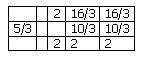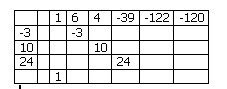## Tuesday, 3 February 2009

### More on Synthetic Division

I wanted to follow up a few things I didn't make as clear as I might have yesterday; first the question about the leading coefficient, then the extension to dividing by a polynomial of higher than the second degree, and the remark about dividing by "breaking up a cubic"

First the question about the leading coefficient.... It doesn't matter what the leading coefficient of the dividend is, but the leading term of the divisor must have a coefficient of one...That is the same as with dividing by a linear factor...... but you can reduce the problem to an equivalent division by dividing out that coefficient...(wow, that was a mouthful.. so here is an example, using the easier linear factor )
Supppose we wanted to divide 6x2 + 16x + 10 by 3x+5.. We need to turn the 3 in 3x+5 into a one, so we divide to get x+5/3.. but this is like simplifying fractions(think of 6x2 + 16x + 10 as the numerator and 3x+5) as the denomiantor) we have to divide both the top and bottom by three to keep the equality, so we also divide 6x2 + 16x + 10 by three, and our new problem is to divide 2x2 + 16/3 x + 10/3 by x + 5/3Keep in mind that if there is a remainder, it will not be the same in the simplified problem as in the original.. If we add 6 to the constant term of the previous problem we get 6x2 + 16x + 16 by 3x+5. Eliminating the leading term of the divisor we get 2x2 + 16/3 x + 16/3 by x + 5/3 and now when we do the synthetic divisionwe get a remainder of 2, instead of six.... ahhh, but we divided both terms by three, so our true remainder is 3x2=6...Keep in mind that one of the things synthetic division does is give us f(2) for a function (the dividend) of x.. If we divide f(x) by three, the value at any point (and hence the remainder on division) is going to be 1/3 of what it would have been otherwise.

And now... the higher degree issue, which seems to work out quite nicely... If we wanted to divide by a cubic we just add three lines, and continue to move the products over one column.. Here is an example dividing x5+ 6x4+4x3-39x2-122x-120 by the cubic x3+3x2-10x-24... note that we still take the negative of each coefficient of the divisor.As we continue we see that the quotient is x2+3x+5 with no remainder...Finally for the cryptic remark about "breaking up a cubic"... In the last problem, if we had wanted to, and if we recognized that x3+3x2-10x-24 could be factored into (x-3)(x2+6x+8), we could have done the last problem in steps. If we divide by one factor and then by the other, we will stil get the same result (although there is still need to pay special attention to remainders). In arithmetic we can divide 24 by 6, or we can divide it by 3, then divide the answer by 2... In the same way we can do the synthetic division in two steps.. I decided (no special reason.. ) to divide by the linear term first.. then the quadratic, as shown here.#### 1 comment:Anonymous said...

Mr. B,
Whats up! hey, love your blogs, teach..but you showed us a way to divide by 3x+5 and stuff that had the remainder right... but there was an extra line?? I have evidence dude.. I kept my notes.. what gives?